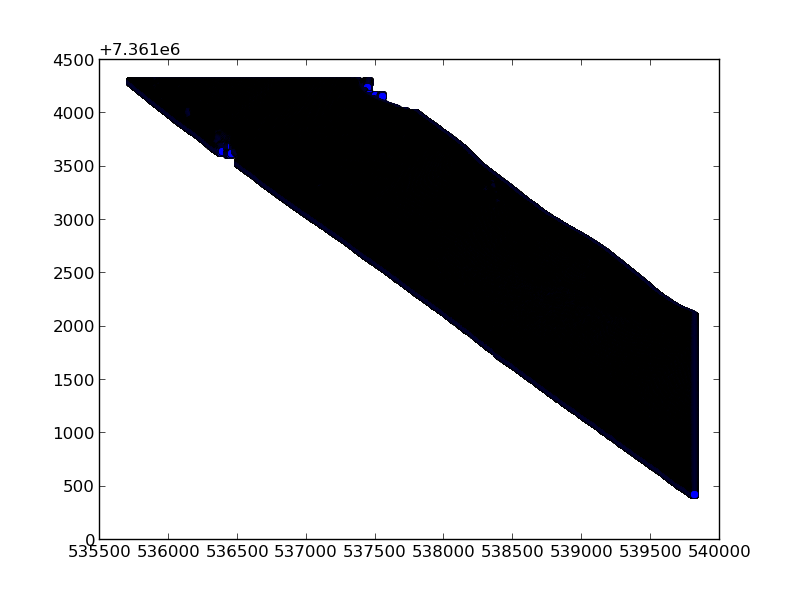# Calculating the convex hull of a point data set (Python)

Working with LiDAR point data it was necessary for me to polygonize the point cloud extent. A first approach was to calculate the convex hull of the points. This is predominantly facilitated using scipy spatial’s ConvexHull function.

For my application I required the hull points to be printed out into a txt/csv in order of position (i.e. going clockwise around the hull). This is enabled by simply pulling out the “vertices” from the spatial.ConvexHull object - this gives the indicies of the convex hull points within the original xy input file:

This is all integrated into a single function:

hull_indicies - which more precisely are the “[i]ndices of points forming the simplical facets of the convex hull” - will look something like this:

The hull vertex indices (hull_indices) can then be passed in with the main xy point file (xy_file) (along with an output path (opath) and output file name (file_name) to the below function which writes out the xy positions of hull_indices from xy_file (in the format I required):

This should give something similar to the following:

Ultimately, from this xy point dataset:I managed to get the following:Written on November 6, 2014